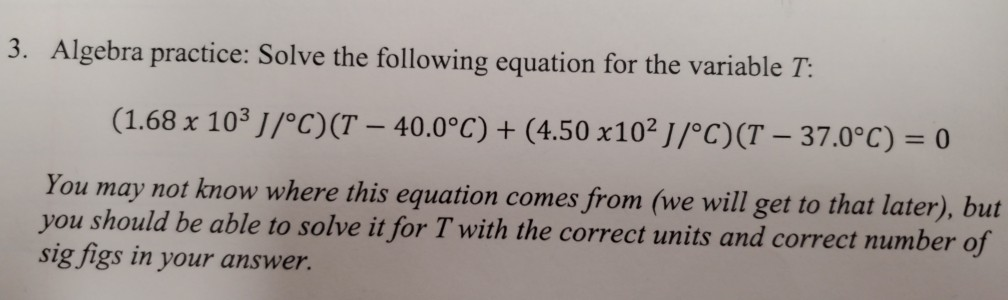# Question Solved1 Answer3. Algebra practice: Solve the following equation for the variable T: (1.68 x 103 J/°C)(T – 40.0°C) + (4.50 x102 1/°C)(T - 37.0°C) = 0 You may not know where this equation comes from (we will get to that later), but you should be able to solve it for T with the correct units and correct number of sig figs in your answer.Transcribed Image Text: 3. Algebra practice: Solve the following equation for the variable T: (1.68 x 103 J/°C)(T – 40.0°C) + (4.50 x102 1/°C)(T - 37.0°C) = 0 You may not know where this equation comes from (we will get to that later), but you should be able to solve it for T with the correct units and correct number of sig figs in your answer.
More
Transcribed Image Text: 3. Algebra practice: Solve the following equation for the variable T: (1.68 x 103 J/°C)(T – 40.0°C) + (4.50 x102 1/°C)(T - 37.0°C) = 0 You may not know where this equation comes from (we will get to that later), but you should be able to solve it for T with the correct units and correct number of sig figs in your answer.